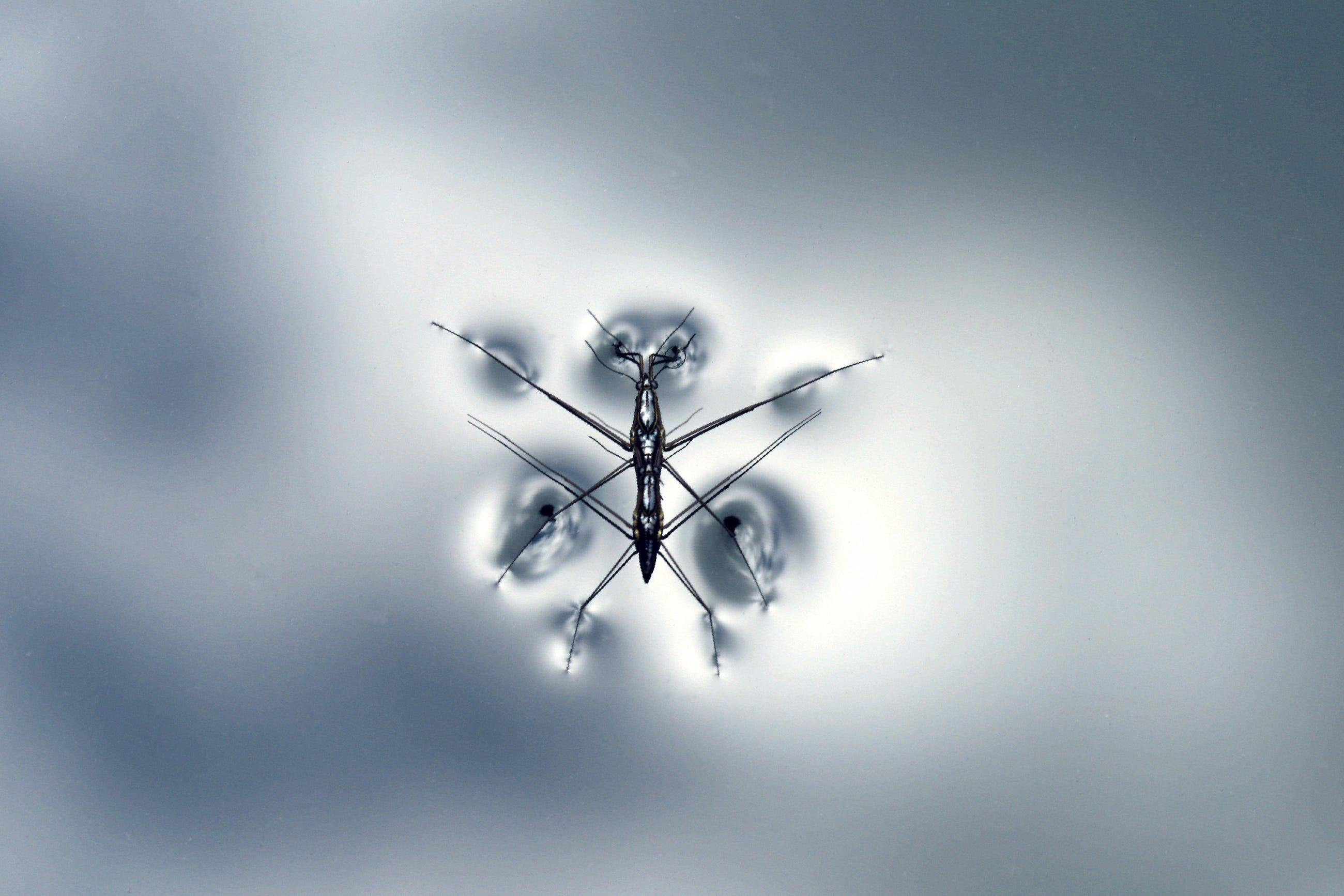# Understanding Convolutional Neural Network with Malaria Cell Image DetectionIn this article, we’ll be learning what is Convolutional Neural Network (CNN) and implement it for Malaria Cell Image dataset. I’ve got the dataset from Kaggle.

CNN is a multilayer perceptron which is good at identifying patterns within datasets. It uses mathematics to extract important features of data to make further classification. As these networks are good with pattern recognition, they are mostly used with images. It could also work with other data but the condition is that data should be in a sequence i.e. shuffling this data must change its meaning.

# Diving deep

To understand CNNs in detail, we need to understand two concepts:

1. Convolutions
2. Pooling

## Convolutions

It is a part of processing the image to read its pattern. This would be the type of layer inside our CNN. It uses a filter matrix to extract the most important features of the image. Most of the time this filter matrix is a grid of 3×3 but it’s possible to change it if need be. It is later multiplied with the image matrix using matrix multiplication. The following diagram is a distinct visualization:

Filters are very useful when dealing with images. You can see more examples of such filters from here. See how these filter values change the aesthetics of the image and highlight particular patterns.

## Pooling

Pooling is used for decreasing the dimensionality of the image. It takes the most important pixels of the image and discards all the other pixels. The below image represents how MaxPooling works in our neural network.

Notice how it decreases the 4×4 matrix to a 2×2 while retaining information of important features.

Further multiple layers of convolutions and pooling are used to get the patterns. This step also helps in decreasing the dimensions for feeding these images to dense layers ahead.

# Into the code

We’ll begin with importing the libraries.

Our dataset contains two folders with different images, parasitized and uninfected. These images should be preprocessed before passing to the model. This step is crucial because it will have a major impact on the accuracy of the model.

For this example, we looped through all the images in the directories and resized every image to 50×50. These images are then added to the data’s list and their respective labels added to the label’s list.

Converting the data into a NumPy array for passing into the model and then shuffling these arrays.

Now, we separate training and testing images. These image arrays are divided by 255 for normalizing the vectors. Wondering why, are you?

Pixels in any image are represented in values between 0 and 255. So dividing the vector with 255 will create values between 0 and 1 which are more normalized and easy for our devices.

## Training the model

A convolutional neural network consists of multiple layers that learn through data stepwise and pass weight to the following layers. It should consist of the layers mentioned below:

1. Conv2D as for convolution layer
2. MaxPooling2D as for decreasing the pixels of image
3. Flatten for converting the result into a flattened array
4. Dense layer with softmax activation for output

We can obviously add other layers if required but this is the standard format used while working with images.

Summary of this model looks like this:

Then, we need to compile our model with loss function, metrics, and optimizer. We’re using adam as optimizer and categorical_crossentropy as loss functions.

Finally, we’ll be fitting the model with training images and labels.

This gives an accuracy of 99.11% at the end of 20 epochs. And gives a test accuracy of 96.11% which is really good. Let’s plot the graphs of accuracy and loss over time.